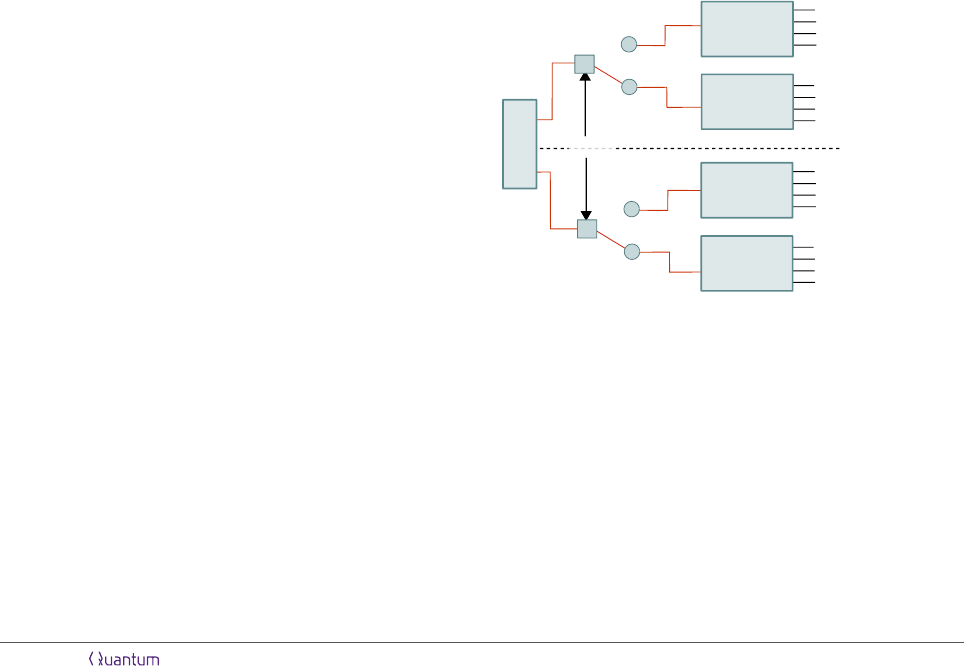René Schwonnek
Institut für Theoretische Physik, Leibniz Universität Hannover, Germany
March 28, 2018
We consider the uncertainty between two
pairs of local projective measurements per-
formed on a multipartite system. We show
that the optimal bound in any linear uncer-
tainty relation, formulated in terms of the
Shannon entropy, is additive. This directly im-
plies, against naive intuition, that the minimal
entropic uncertainty can always be realized by
fully separable states. Hence, in contradic-
tion to proposals by other authors, no entan-
glement witness can be constructed solely by
comparing the attainable uncertainties of en-
tangled and separable states. However, our re-
sult gives rise to a huge simpliﬁcation for com-
puting global uncertainty bounds as they now
can be deduced from local ones.
Furthermore, we provide the natural gener-
alization of the Maassen and Uﬃnk inequality
for linear uncertainty relations with arbitrary
positive coeﬃcients.
Introduction
Uncertainty and entanglement are doubtless two of
the most prominent and drastic properties that set
apart quantum physics from a classical view on the
world. Their interplay contains a rich structure,
which is neither suﬃciently understood nor fully dis-
covered. In this work, we reveal a new aspect of this
structure: the additivity of entropic uncertainty rela-
tions.
For product measurements in a multipartition, we
show that the optimal bound c
ABC...
in a linear un-
certainty relation satisﬁes
c
ABC···
= c
A
+ c
B
+ c
C
+ . . . , (1)
where c
A
, c
B
, c
C
, . . . are bounds that only depend on
local measurements. This result implies that minimal
uncertainty for product measurements can always be
realized by uncorrelated states. Hence, we have an
example for a task which is not improved by the use
of entanglement.
We will quantify the uncertainty of a measurement
by the Shannon entropy of its outcome distribution.
For this case, the corresponding linear uncertainty
bound c
ABC...
gives the central estimate in many ap-
plications like: entropic steering witnesses , un-
certainty relations with side-information , some se-
curity proofs  and many more.
When speaking about uncertainty, we consider so
called preparation uncertainty relations . From
an operational point of view, a preparation uncer-
tainty describes fundamental limitations, i.e. a trade-
oﬀ, on the certainty of predicting outcomes of sev-
eral measurements that are performed on instances
of the same state. This should not be confused 
with its operational counterpart named measurement
uncertainty. A measurement uncertainty rela-
tion describes the ability of producing a measurement
device which approximates several incompatible mea-
surement devices in one shot.
The calculations in this work focus on uncertainty
relations in a bipartite setting. However, all results
can easily be generalized to a multipartite setting by
an iteration of statements on bipartitions. The ba-
sic measurement setting, which we consider for bi-
partitions, is depicted in Fig. 1. We consider a pair
ρ
AB
X
A
Y
A
{1, 0}
1
0
X
B
Y
B
1
0
A
B
λ p
X
AB
µ p
Y
AB
Figure 1: Basic setting of product measurements on a biparti-
tion: pairs of measurements X
A
, X
B
or Y
A
, Y
B
are applied to
a joint state ρ
AB
at the respective sides of a bipartition. One
bit of information is transmitted for communicating whether
the X or the Y measurements are performed. The weights
(λ, µ) denote the probabilities corresponding to this choice.
of measurements, X
AB
= X
A
X
B
and Y
AB
= Y
A
Y
B
,
to which we will refer as the global measurements of
(tensor) product form. Each of those global measure-
ments of product form is implemented by applying
local measurements at the respective sides of a bipar-
tition between parties denoted by A and B. Hereby,
Accepted in Quantum 2018-03-20, click title to verify 1
arXiv:1801.04602v4 [quant-ph] 27 Mar 2018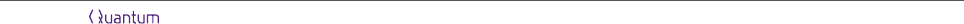the variables X
A
, X
B
and Y
A
, Y
B
will refer to those
local measurements applied to the respective sides.
We only consider projective measurements, but be-
side this we impose no further restrictions on the in-
dividual measurements. So the only property that
measurements like X
A
and X
B
have to share is the
common label X’, besides this, they could be non-
commuting or even deﬁned on Hilbert spaces with
diﬀerent dimensions.
The main result of this work is stated in Prop.1 in
Sec. 3. In that section, we also collect some remarks
on possible and impossible generalizations and the
construction of entanglement witnesses. The proof of
Prop.1 is placed at the end of this paper, as it relies
on two basic theorems stated in Sec.4 and Sec.5.
Thm.1, in Sec.4, clariﬁes and expands the known
connection between the logarithm of (p, q)-norms and
entropic uncertainty relations. As a special case of
this theorem we obtain Lem.1 which states the nat-
ural generalization of the well known Maassen and
Uﬃnk bound  to weighted uncertainty relations.
Thm.2, in Sec.5, states that (p, q)-norms, in a certain
parameter range, are multiplicative, which at the end
uncertainty relations.
Before stating the main result, we collect, in Sec.1,
some general observations on the behavior of uncer-
tainty relations for product measurements with re-
spect to diﬀerent classes of correlated states. Fur-
thermore, in Sec. 2, we will motivate and explain the
explicit form of linear uncertainty relations used in
this work.
1 Uncertainty in bipartitions
All uncertainty relations considered is this paper
are state-independent. In practice, ﬁnding a state-
independent relation leads to the problem of jointly
minimizing a tuple of given uncertainty measures,
here the Shannon entropy of X
AB
and Y
AB
, over all
states. This minimum, or a lower bound on it, then
gives the aforementioned trade-oﬀ, which then allows
to formulate statements like: "whenever the uncer-
tainty of X
AB
is small, the uncertainty of Y
AB
has to
be bigger than some state-independent constant" .
Considering the measured state, ρ
AB
, it is natural
to distinguish between the three classes: uncorrelated,
classically correlated and non-classical correlated. In
regard of the uncertainty in a corresponding global
measurement, states in these classes share some com-
mon features:
If the measured state is uncorrelated, i.e a prod-
uct state ρ
AB
= ρ
A
ρ
B
, the outcomes of the lo-
cal measurements are uncorrelated as well. Hence,
the uncertainty of a global measurement is completely
determined by the uncertainty of the local measure-
ments on the respective local states ρ
A
and ρ
B
. More-
over, in our case, the additivity of the Shannon en-
tropy, tells us that the uncertainty of a global mea-
surement is simply the sum of the uncertainty of the
local ones. In the same way any trade-oﬀ on the global
uncertainties can be deduced from local ones.
If the measured state is classically correlated,
i.e a convex combination of product states , ad-
ditivity of local uncertainties does not longer hold.
More generally, whenever we consider a concave un-
certainty measure , like the Shannon entropy, the
global uncertainty of a single global measurement is
smaller than the sum of the local uncertainties. Intu-
itively this makes sense because a correlation allows
to deduce information on the potential measurement
outcomes of one side given a particular measurement
outcome on the other. However, a linear uncertainty
relation for a pair of global measurements is not af-
fected by this, i.e a trade-oﬀ will again be saturated
by product states. This is because the uncertainty re-
lation between two measurements, restricted to some
convex set of states, will always be attained on an
extreme point of this set.
However, if measurements are applied to an entan-
gled state, more precisely to a state which shows
EPR-steering  with respect to the measure-
ments X
AB
and Y
AB
, it is in general not clear how
a trade-oﬀ between global uncertainties relates to the
corresponding trade-oﬀ between local ones. Just have
in mind that steering implies the absence of any lo-
cal state model, which is usually proven by showing
that any such model would violate a local uncertainty
relation.
In principle one would expect to obtain smaller un-
certainty bounds by also considering entangled states,
and there are many entanglement witnesses known
section).
2 Linear uncertainty relations
We note that there are many uncertainty measures,
most prominently variances [8, 10]. Variance, and
similar constructed measures [17, 27], describe the
deviation from a mean value, which clearly demands
to assign a metric structure to the set of measure-
ment outcomes. From a physicist’s perspective this
makes sense in many situations  but can also cause
strange behaviours in situations where this metric
structure has to be imposed artiﬁcially . However,
from the perspective of information theory, this seems
to be an unnecessary dependency. Especially when
uncertainties with respect to multipartitions are con-
sidered, it is not clear at all how such a metric should
be constructed. Hence, it can be dropped and a quan-
tity that only depends on probability distributions of
measurement outcomes has to be used. We will use
the Shannon entropy. It fulﬁlls the above require-
ment, does not change when the labeling of the mea-
surement outcomes are permuted, and has a clear op-
Accepted in Quantum 2018-03-20, click title to verify 2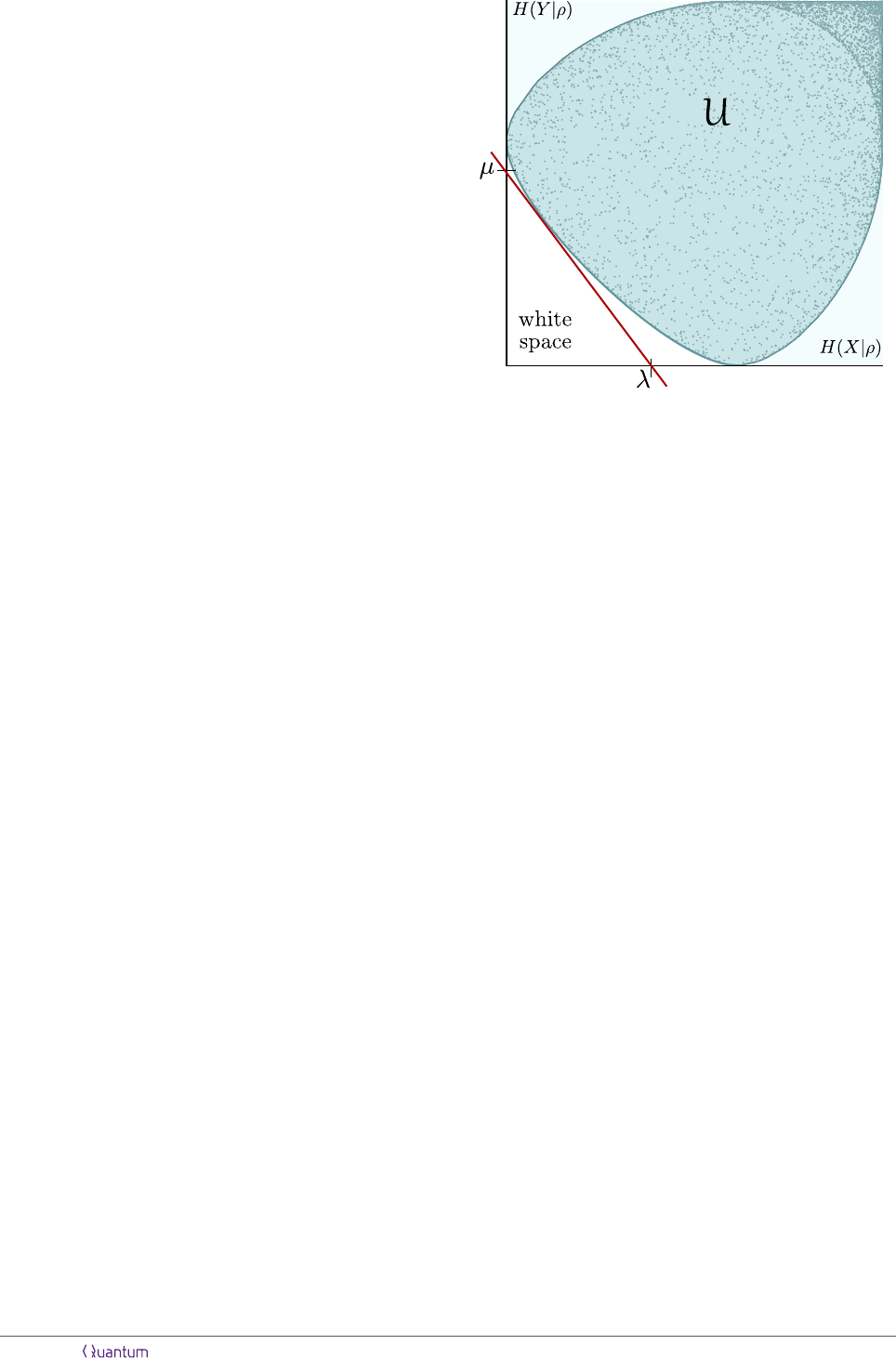erational interpretation [29, 30]. Remarkably, Claude
Shannon himself used the term ’uncertainty’ as an in-
tuitive paraphrase for the quantity today known as
’entropy’ . Historically, the decision to call the
Shannon entropy an entropy’ goes back to a sugges-
tion John von Neumann gave to Shannon, when he
was visiting Weyl in 1940 (there are, at least, three
versions of this anecdote known , the most popular
is ).
Because we are not interested in assigning values
to measurement outcomes, a measurement, say X, is
suﬃciently described by its POVM elements, {X
i
}.
So, given a state ρ, the probability of obtaining the
i-th outcome is computed by tr(ρX
i
). The respec-
tive probability distribution of all outcomes is de-
noted by the vector p
x
ρ
. Within this notation the
Shannon entropy of a X measurement is given by
H(X|ρ) :=
P
i
p
x
ρ
i
log
p
x
ρ
i
. As we restrict our-
selves to non-degenerate projective measurements, all
necessary information on a pair of measurements, X
and Y , is captured by a unitary U that links the mea-
surement basis. We will use the convention to write
U as transformation from the {X
i
} to the {Y
i
}-basis,
i.e. we will take U such that Y
i
= UX
i
U
holds.
Our basic objects of interest are optimal, state-
independent and linear relations. This is, for ﬁxed
weights λ, µ R
+
we are interested in the best con-
stant c(λ, µ) for which the linear inequality
λH(X|ρ) + µH(Y |ρ) c(λ, µ) (2)
holds on all states ρ.
Such a relation has two common interpretations:
On one hand one can consider a guessing game, see
also . On the other, a relation like (2) can be
interpreted geometrically as in Fig. 2.
Linear uncertainty: a guessing game
For the moment, consider a player, called Eve, who
plays against an opponent, called Alice. Depen-
dent on a coin throw, in each round, Alice performs
measurement X
A
or Y
A
on a local quantum state.
Thereby the weights λ and µ are the weights of the
coin and the l.h.s. of (2) describes the total uncer-
tainty Eve has on Alice’s outcomes in each round. To
be more precise, up to a (λ, µ)-dependent constant,
the l.h.s of (2) equals the Shannon entropy of the
outcome distribution λp
X
A
ρ
µp
Y
A
ρ
.
Eve’s role in this game is to ﬁrst choose a state ρ,
observe the coin throw, wait for the measurements
to be performed by Alice, and then ask binary ques-
tions to her opponent in order to get certainty on the
outcomes. Thereby, the Shannon entropy sum on the
l.h.s of (2) (with logarithm to the base 2) equals the
expected amount questions Eve has to ask using an
optimal strategy based on a ﬁxed ρ. Hence, the value
c(λ, µ) denotes the minimal amount of expected ques-
tions, attainable by choosing an optimal ρ.
For a bipartite setting, Fig. 1, a second player,
say Bob, joins the game. Here, Eve will play the
Figure 2: Uncertainty set for measurements performed on
a qubit. Any linear uncertainty relation, (2), with weights
(λ, µ), gives the description of a tangent to the uncertainty
set. All attainable pairs of entropies lie above this tangent.
above game against Alice and Bob, simultaneously.
Thereby, Alice and Bob share a common coin, and,
therefore, apply measurements with the same labels
(X
AB
or Y
AB
). The obvious question that arises in
this context is if Eve gets an advantage in this si-
multaneous game by using an entangled state or not.
Prop. 1 in the next section answers the above ques-
tion negatively, which is somehow unexpected as in
principle the possible usage of non-classical correla-
tions enlarges Eve’s strategies. For example: Eve
could have used a maximally entangled state, adjusted
such that all measurements Alice and Bob perform
are maximally correlated. In this case the remaining
uncertainty Eve has, would only be the uncertainty
on the outcomes of one of the parties. However, the
marginals of a maximally entangled state are maxi-
mally mixed. Hence, Eve still has a serious amount of
uncertainty (log d), which turns out to be not small
enough for beating a strategy based on minimizing
the uncertainty of the local measurements individu-
ally. For the case of product-MUBs in prime square
dimension , it turns out that the minimal uncer-
tainty realizable by a maximally entangled state ac-
tually equals the optimal bound.
Linear uncertainty: the positive convex hull
The second interpretation comes from considering the
set of all attainable uncertainty pairs, the so called
uncertainty set
U = {(H(X|ρ), H(Y |ρ)) |ρ is a quantum state} . (3)
In principle this set contains all information on the un-
certainty trade-oﬀ between two measurements. More
precisely, the white space in the lower-left corner of
a diagram like Fig. 2 indicates that both uncertain-
Accepted in Quantum 2018-03-20, click title to verify 3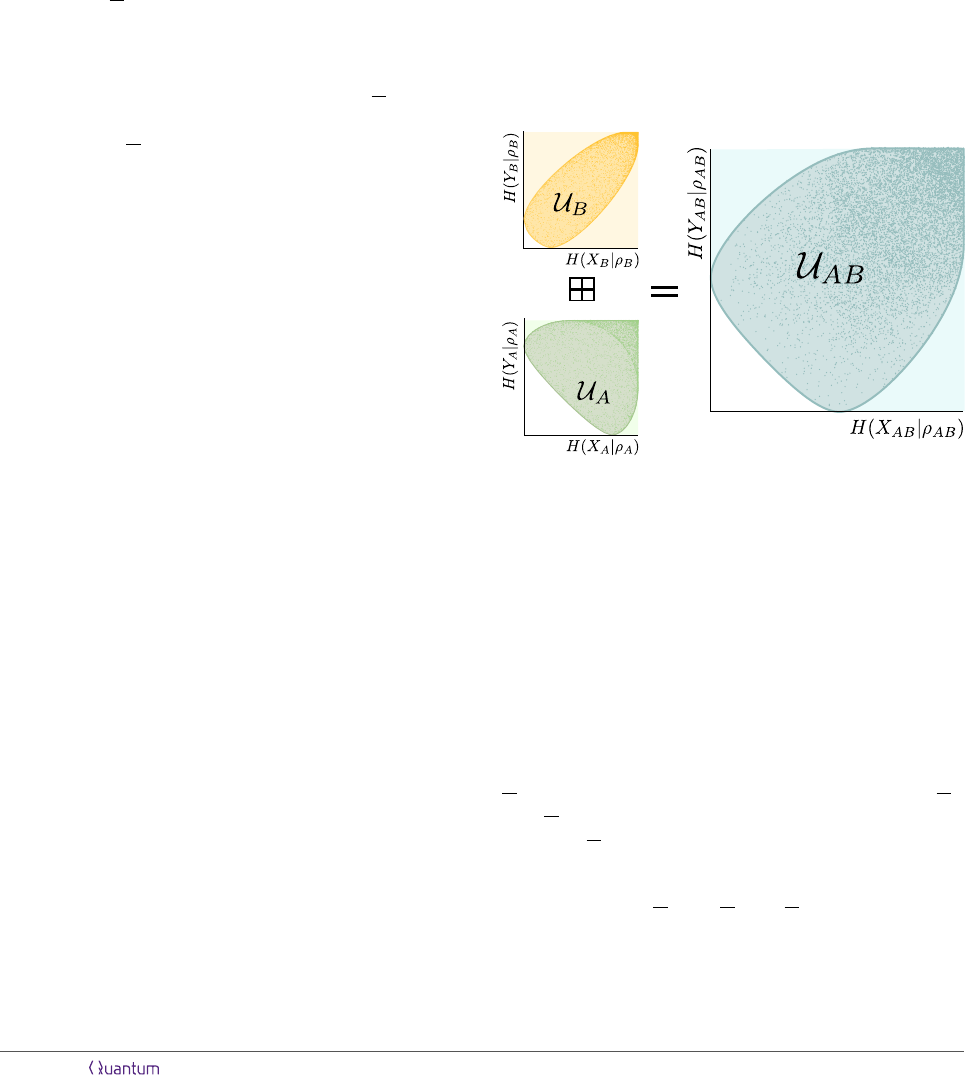ties cannot be small simultaneously. In this context, a
state-independent uncertainty gives a quantitative de-
scription of this white space. Unfortunately, it turns
out that computing U can be very hard, because the
whole state-space has to be considered. Here a lin-
ear inequality, like (2), gives an outer approximation
of this set. More precisely, if c(λ, µ) is the optimal
constant in (2), this inequality describes a halfspace
bounded from the lower-left by a tangent on U. This
tangent has the slope µ/λ. The points on which this
tangent touches the boundary of U corresponds to
states which realize equality in (2). Those states are
called minimal-uncertainty states. Given all those
tangents, i.e. c(λ, µ) for all positive (λ, µ), we can
intersect all corresponding halfspaces and get a con-
vex set which we call the positive convex hull of U,
denoted by
U in the following. Geometrically, the
positive convex hull can be constructed by taking the
convex hull of U and adding to it all points that have
bigger uncertainties then, at least, some point in U.
If U is convex, like in the example above, U contains
the full information on the relevant parts of U. If U
is not convex, U still gives a variety of state indepen-
dent uncertainty relations, but there is still place for
ﬁnding improvements, see .
tions
We are now able to state our main result
Proposition 1 (Additivity of linear uncertainty rela-
tions). Let c
A
(λ, µ) and c
B
(λ, µ) be state-independent
lower bounds on the linear entropic uncertainty for lo-
cal measurements X
A
, X
B
and Y
A
, Y
B
, with weights
(λ, µ). This means we have that
λH(X
A
|ρ
A
) + µH(Y
A
|ρ
A
) c
A
(λ, µ)
λH(X
B
|ρ
B
) + µH(Y
B
|ρ
B
) c
B
(λ, µ) (4)
holds on any state ρ
A
from B(H
A
) and ρ
B
from
B(H
B
). Let X
AB
and Y
AB
be the joint global mea-
surements that arise from locally performing X
A
, X
B
and Y
A
, Y
B
respectively. Then
λH(X
AB
|ρ
AB
) + µH(Y
AB
|ρ
AB
) c
A
(λ, µ) + c
B
(λ, µ)
(5)
holds for all states ρ
AB
from B(H
A
H
B
). Further-
more, if c
A
and c
B
are optimal bounds, then
c
AB
(λ, µ) := c
A
(λ, µ) + c
B
(λ, µ) (6)
is the optimal bound in (5), i.e. linear entropic un-
The proof of this proposition is placed at the end
of Sec. 5. We will proceed this section by collecting
some remarks related to the above proposition:
Remark 1 (Product states). Assume that c
A
(λ, µ)
and c
B
(λ, µ) are optimal constants, and φ
A
and φ
B
are the states that saturate the corresponding uncer-
tainty relations (4). Then the product state φ
AB
:=
φ
A
φ
B
saturates (5), due to the additivity of the
Shannon-entropy. However, this does not imply that
all states that saturate (4) have to be product states.
Examples for this, involving MUBs of product form,
are provided in .
Remark 2 (Minkowski sums of uncertainty regions).
Prop. 1 shows how the uncertainty set U
AB
, of the
product measurement, relates to the uncertainty sets
U
A
and U
B
of corresponding local measurements: For
the case of an optimal c
AB
(λ, µ), and ﬁxed (λ, µ),
equality in (5) can always be realized by product
states (see Rem. 1). In an uncertainty diagram, like
Fig. 3, those states correspond to points on the lower-
left boundary of an uncertainty set, and, in general,
they produce the ﬁnite extreme points of the positive
convex hull of an uncertainty set.
Figure 3: Uncertainty sets of local measurements can be
combined by the Minkowski sum: Uncertainty sets (green and
yellow) for two pairs of local measurements on Qubits and
the uncertainty set of the corresponding global measurements
(blue).
For product states we have the additivity of the
Shannon entropy, which gives
H(X
AB
|φ
A
φ
B
)
H(Y
AB
|φ
A
φ
B
)
=
H(X
A
|φ
A
)
H(Y
A
|φ
A
)
+
H(X
B
|φ
B
)
H(Y
B
|φ
B
)
(7)
This implies that we can get every extreme point of
U
AB
by taking the sum of two extreme points of U
A
and U
B
. Due to convexity the same holds for all
points in U
AB
and we can get this set as Minkowski
sum .
U
AB
= U
AB
U
B
(8)
For convex uncertainty regions, arising from local
measurements, this is depicted in Fig. 3. For this
example, it is also true that U
AB
itself is given as
Accepted in Quantum 2018-03-20, click title to verify 4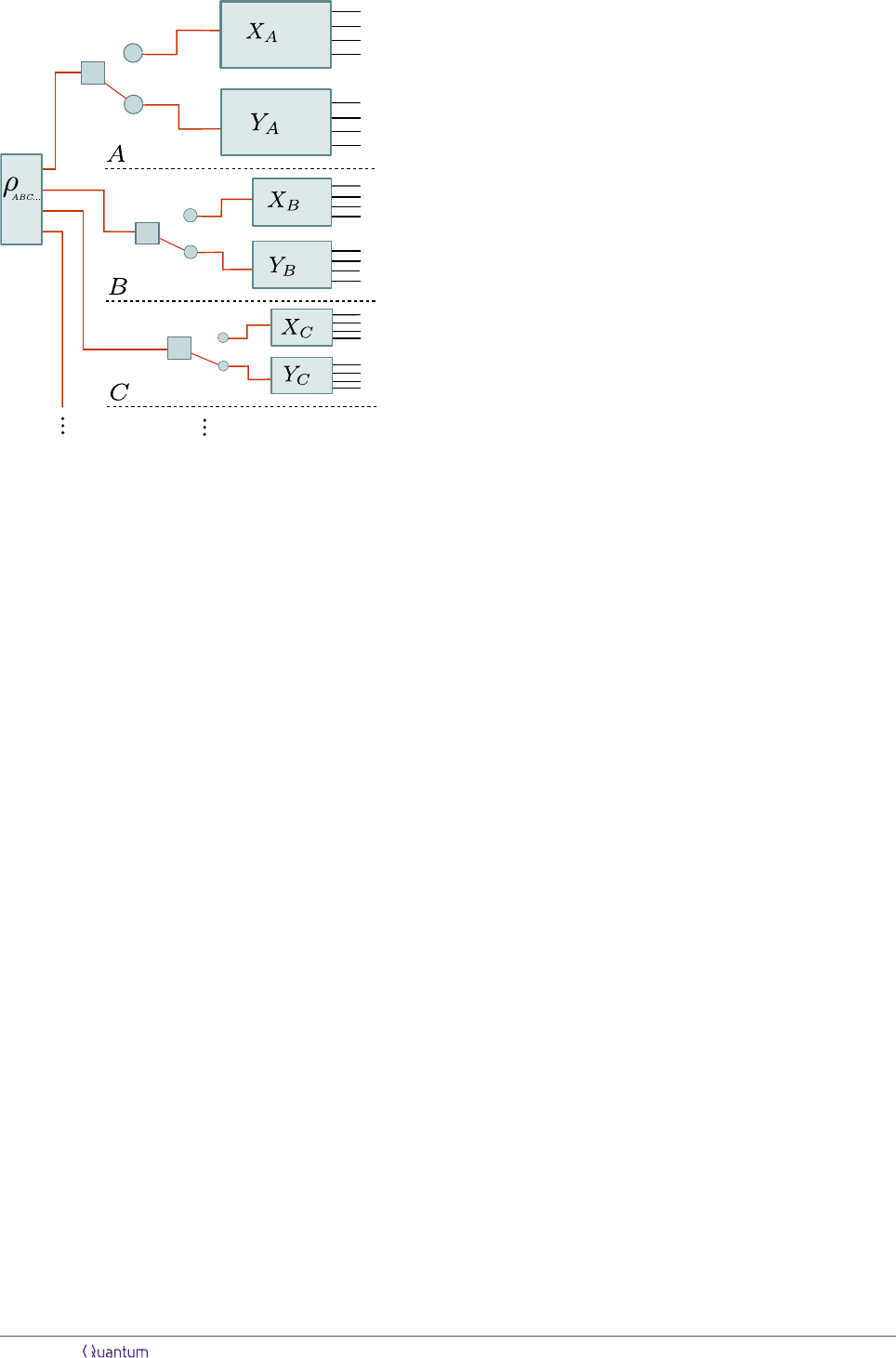Figure 4: Multiparite setting: Additivity of entropic uncer-
tainty relations also holds if a pair of global product mea-
surements for many local parties is considered.
Minkowski sum of local uncertainty sets. However,
we have to note, this behavior cannot be concluded
from Prop. 1 alone.
Remark 3 (Relation to existing entanglement wit-
nesses). A well know method for constructing non-
linear entanglement witnesses is based on computing
the minimal value of a functional, like the sum of
uncertainties , attainable on separable states.
Given an unknown quantum state, the value of this
functional is measured. If the measured value under-
goes the limit set by separable states, the presence of
entanglement is witnessed. For uncertainty relations
based on the sum of general Schur concave functionals
this method was proposed in , including Shannon
entropy, i.e. the l.h.s. of (5), as central example.
Our result Prop. 1 shows that this method will not
work for Shannon entropies, because there is no en-
tangled state that undergoes the limit set by separable
states. We note that there is no mathematical contra-
diction between Prop. 1 and . We only show that
the set of examples for the method proposed in  is
empty.
For uncertainty relations in terms of Shannon, Tsal-
lis and Renyi entropies a similar procedure for con-
structing witnesses was proposed by [37, 39]. Here
explicit examples for states, that can be witnessed to
be entangled, were provided. Again, our proposition
Prop. 1 is not in contradiction to this work because
in [37, 39] observables with a non-local degeneracy
where considered.
Prop. 1 can easily be generalized to a multipartite
setting, see Fig. 4 :
Corollary 1 (Generalization to multipartite mea-
surements). Assume parties A
1
. . . A
n
that lo-
cally perform measurements, X
A
1
, . . . , X
A
n
or
Y
A
1
, . . . , Y
A
n
, with weights
~
λ = (λ
1
, . . . , λ
n
). In anal-
ogy to (4), let c
A
1
(
~
λ), . . . , c
A
n
(
~
λ) denote optimal lo-
cal bounds and let c
A
1
···A
n
(
~
λ) be the optimal bound
corresponding to product measurements X
A
1
...A
n
and
Y
A
1
...A
n
. We have
c
A
1
...A
n
(
~
λ) =
n
X
i=1
c
A
i
(
~
λ) (9)
This follows by iterating (6).
Remark 4 (Generalization to three measurements).
The generalization of Prop. 1 to three measurements,
say X
AB
, Y
AB
and Z
AB
, fails in general. The follow-
ing counterexample was provided by O. Gühne :
For both parties we consider local measurements de-
duced from the three Pauli operators on a qubit and
take all weights equal to one. In short hand nota-
tion we write X
AB
= σ
X
σ
X
, Y
AB
= σ
Y
σ
Y
, and
Z
AB
= σ
Z
σ
Z
. In this case, the minimal local un-
certainty sum is attained on eigenstates of the Pauli
operators. If such a state is measured, the entropy
for one of the measurements is zero and maximal for
the others. Hence, the local uncertainty sum is always
bigger than 2 [bit]. Therefore we have
H (σ
X
σ
X
|φ
A
φ
B
) +
H (σ
Y
σ
Y
|φ
A
φ
B
) +
H (σ
Z
σ
Z
|φ
A
φ
B
) 4 (10)
for all product states. In contrast to this a Bell state,
say Ψ
, will give the entropy of 1[bit], for all above
measurements. Hence we have,
H
σ
X
σ
X
|Ψ
+
H
σ
Y
σ
Y
|Ψ
+
H
σ
Z
σ
Z
|Ψ
= 3 4. (11)
4 Lower bounds from (p, q)-norms
The quite standard technique for analyzing a linear
uncertainty relation is to connect it to the (p, q)-
norm (see (12) below) of the basis transformation U.
Thereby, the majority of previous works in this ﬁeld
is concentrating only on handling the case of equal
weights λ = µ = 1, which is connected to the (p, q)-
norm for the case 1/p + 1/q = 1. However, for the
purpose of this work, i.e. for proving Prop. 1, we have
to extend this connection to arbitrary (λ, µ). We will
do this by Thm. 1 on the next page.
A historically important example for the use of the
connection between (p, q)-norms and entropic uncer-
tainties, is provided by Bialynicki-Birula and Myciel-
ski . They used Beckner’s result , who com-
puted the (p, q)-norm of the Fourier-Transfromation,
Accepted in Quantum 2018-03-20, click title to verify 5for proving the corresponding uncertainty relation,
between position and momentum, conjectured by
Hirschmann . Also Maassen and Uﬃnk  took
this way for proving their famous relation. Our result
gives a direct generalization of this, meaning we will
recover the Maassen and Uﬃnk relation at the end
of this section as special case of (50). Albeit, before
stating our result, we will start this section by shortly
reviewing the previously known way for connecting
[44, 45] for further details:
The (p, q)-norm, i.e the l
p
l
q
operator norm, of
a basis transformation U is given by
kUk
q,p
:= sup
φ∈H
kUφk
q
kφk
p
. (12)
Here, the limit of kU k
q,p
for (p, q) (2, 2) goes to
1. However, when p and q are ﬁxed on the curve
1/p + 1/q = 1, the leading order of kUk
q,p
around
(p, q) = (2, 2) recovers the uncertainty relation (2) in
the case of equal weights λ = µ = 1/2, see [41, 43].
More precisely, taking the negative logarithm of
(12) gives
log kUk
q,p
= inf
φ∈H
log kφk
p
log kUφk
q
. (13)
Here, we can identify the squared modulus of the com-
ponents of φ as probabilities of the X and Y measure-
ment outcomes
|(φ)
i
|
2
= hφ| X
i
|φi = (p
X
φ
)
i
|(Uφ)
i
|
2
= hφ| Y
i
|φi = (p
Y
φ
)
i
(14)
and substitute
kφk
p
=
kp
X
φ
k
p/2
2
and kUφk
q
=
kp
Y
φ
k
q/2
2
.
(15)
By this, (13) gives a linear relation in terms of the
α-Renyi entropy , H
α
(p) =
α
1α
log(kpk
α
). Here
we get
inf
φ∈H
2 p
p
H
p/2
(X|φ)
2 q
q
H
q/2
(Y |φ) = log kUk
2
q,p
.
(16)
If we evaluate this on the curve 1/p + 1/q = 1, for
p 2 q, we can use
2 p
p
=
1
p
1
q
=
q 2
q
, (17)
which can be employed to (16), in order to get
inf
φ∈H
H
p/2
(X|φ) + H
q/2
(Y |φ) =
1
q
1
p
1
log kUk
2
q,p
.
(18)
Here, the limit (p, q) (2, 2), in the l.h.s of (18),
gives the limit from the Renyi to the Shannon en-
tropy. This gives the l.h.s. of the uncertainty relation
(2) for λ = µ = 1. Hence, the functional depen-
dence of kUk
q,p
on (p, q) in the limit (p, q) (2, 2)
gives the optimal bound c(1, 1), in (2). For the
case of the L
2
(R)-Fourier transformation the norm
kU
F
k
q,p
=
p
p
1/p
/
p
q
1/q
was computed by Beckner
, leading to c(1, 1) = log(πe). However, to the
best of our knowledge, computing kUk
q,p
, for general
U and (p, q), is an outstanding problem, and presum-
ably very hard [47, 48]. Albeit, for special choices of
(p, q) this problem gets treatable, see  for a list of
those. The known cases include p = q = 2, p = or
q = such as p = 1 or q = 1.
The central idea of Maassen’s and Uﬃnk’s work 
is to show that the easy case of (p = 1, q = ), here
we have kUk
1,
= max
ij
|U
ij
|, gives a lower bound on
c(1, 1). More precisely, they show that, for 1 p 2
and on the line 1/p + 1/q = 1, the r.h.s. of (18)
approaches c(1, 1) from below. Note that this is far
from being obvious. Explicitly, for p 2 q we have
H
q/2
(Y |φ) H(Y |φ) and H
p/2
(X|φ) H(X|φ),
so one term approaches the limit from above and
the other approaches the limit from below. Whereas
Maassen and Uﬃnk showed, using the Riesz-Thorin
interpolation [50, 51], that the inf
φ
of the sum of both
approaches the limit from below.
The following Theorem, Thm.1, extends the above
to the case of arbitrary (λ, µ). Notably, we have to
take (p, q) from curves with 1/p + 1/q 6= 1, those are
depicted in Fig. 5. In contrast to Maassen and Uﬃnk,
the central inequality we use is the -norm versions of
the Golden Thompson inequality (see  and the
blog of T.Tao  for a proof and related discussions).
Theorem 1. Let c(λ, µ), with λ, µ R
+
, be the op-
timal constant in the linear weighted entropic uncer-
tainty relation
c(λ, µ) := inf
ρ
λH (X|ρ) + µH (Y |ρ) . (19)
Then:
(i) c(λ, µ) is bounded from below by N log (ω
N
(λ, µ))
with ω
N
(λ, µ) = sup
xB
r
(C
d
)
yB
s
(C
d
)
x
Uy
(20)
and r =
2N
N + 2λ
s =
2N
N + 2µ
(21)
where
B
r
(Ω) := {x | 1 kxk
r
}
denotes the unit r-norm Ball on .
(ii) For λ, µ N/2 we can write
ω
N
(λ, µ) = sup
φC
d
kUφk
r
0
kφk
s
= sup
φC
d
kUφk
s
0
kφk
r
(22)
with r
0
=
2N
N 2λ
s
0
=
2N
N 2µ
(23)
(24)
Accepted in Quantum 2018-03-20, click title to verify 6